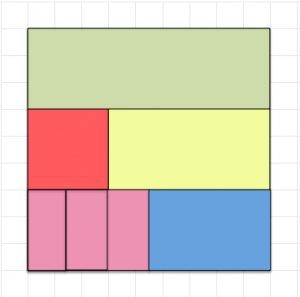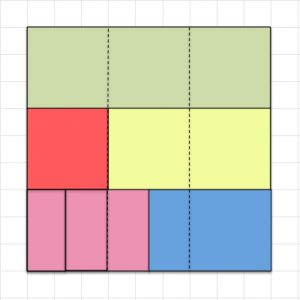This square is one unit and it is split into smaller parts. Label each part with the fraction it shows.

How many green rectangles make one unit?

How many small red squares make one unit?

How many small blue rectangles make one unit?

How many small pink rectangles make one unit?

Which coloured bits would you use to show that three eighteenths equals one sixth? $\frac{3}{18}=\frac{1}{6}$

Which coloured bits would you use to show that two sixths equals one third? $\frac{2}{6}=\frac{1}{3}$

Which coloured bits would you use to show that one ninth plus two ninths equals one third? $\frac{1}{9}+\frac{2}{9}=\frac{1}{3}$. Why does this addition work that way?

You may find that this 3 by 3 grid helps you to answer the questions.

Draw your own patternin a square and label the fractions in your pattern.